﻿ Controllers Tuning through Multi-objective Non-Dominated Sorting Genetic Algorithms

Controllers Tuning through Multi-objective Non-Dominated Sorting Genetic Algorithms

CHOURAQUI Samira, BENZATER HabibaOPEN ACCESSPEER-REVIEWED

Controllers Tuning through Multi-objective Non-Dominated Sorting Genetic Algorithms

CHOURAQUI Samira1,, BENZATER Habiba1

1Department of Computer Science, University of Sciences and Technology USTO’MB, El Mnouar, Algeria

Abstract

In control system design there are often a number of design objectives to be considered. The objectives are sometimes connecting and no design exists which can be considered best with respect to all objectives. Hence, there is an inevitable tradeoff between design objectives, for example, between and output performance objective and stability robustness. These considerations have led to the study of multi objective optimization methods for control systems. In this paper a multi-objective Non-Dominated sorting genetic Algorithms NSGA-II is used to tuning of Proportional Derivative (PD) controller of a six freedom arm manipulator PUMA560. The NSGAII algorithm searches for the controller PD gains so that the six values of Integral Absolute Error (IAE) in joint space are minimized. Simulation numerical results of multivariable PD control and convergence of the NSGA-II are presented and discussed.

At a glance: Figures

• Samira, CHOURAQUI, and BENZATER Habiba. "Controllers Tuning through Multi-objective Non-Dominated Sorting Genetic Algorithms." World Journal Control Science and Engineering 1.1 (2013): 15-24.
• Samira, C. , & Habiba, B. (2013). Controllers Tuning through Multi-objective Non-Dominated Sorting Genetic Algorithms. World Journal Control Science and Engineering, 1(1), 15-24.
• Samira, CHOURAQUI, and BENZATER Habiba. "Controllers Tuning through Multi-objective Non-Dominated Sorting Genetic Algorithms." World Journal Control Science and Engineering 1, no. 1 (2013): 15-24.

 Import into BibTeX Import into EndNote Import into RefMan Import into RefWorks

1. Introduction

In control system design there are often a number of design objectives to be considered. The objectives are sometimes conflicting and no design exists which can be considered best with respect to all objectives. Hence, there is an inevitable tradeoff between design objectives, for example, between output performance objective and stability robustness. These considerations have led to the study of multi objective optimization methods for control systems.

Although there has been considerably more research into multi objective decision making in the field of systems engineering than into multi objective optimization design of multivariable control, more and more research in multi objective control has been carried out. The main research areas are multi objective robust control [1, 2], multi objective critical control , multi objective eigenstructure assignment , multi objective PID control [5, 6, 7], multi objective identification , multi objective fault detection , and multi objective linear quadratic Gaussian control [10, 11, 12, 13, 14].

In this paper a we use the improved non-dominated sorting genetic algorithm (NSGA-II), which is one of the most powerful non aggregative multi-objective techniques, and able to locate the Pareto front in complex reach space. The design of PD controller is considered here and it formulated as multi-objective optimization problem where the integral absolute error is optimized simultaneously. The remainder of the paper is organized as follows:

In section 2, a general description of the multi-objective optimization algorithm is presented. Dynamic formulation of robot manipulator is presented in section 3. In section 4, NSGA-II algorithm tuning PD gains is presented. The simulation results of the model and the NSGAII algorithm are shown in section 5. A conclusion follows in section 6.

2. Multi-objective Optimization Algorithm

The optimization problem is minimized or maximized an objective function. In single-objective optimization, it is possible to determine between any given pair of solutions if one is better than the other, as result the best single solution is the goal. However, multi-objective optimization (MOO) problems in which the designer seeks to optimize simultaneously several objectives, there is usually no single optimal solution .

The method most commonly adopted to compare solutions in multi-objective optimization is called Pareto dominance relation which, instead of a single optimal solution, leads to a set of solutions. These solutions are called Pareto optimal solutions or non-dominated solutions. The Pareto set consists of solutions that are not dominated by any other solutions. A solution x is said to dominate y if x is better or equal to y in all attributes, and strictly better in at least one attribute .

Figure 1 shows a classification of the methods used to solve an MOO problem in literature given in .

Multi-Objective Evolutionary Optimization (MOEA) is an approach useful that offers an alternate means of solving multi-objective optimization problems compared to classical approaches. Since evolutionary algorithms use a population based approach, they allow an efficient way to find an approximation of the whole Pareto front in a single simulation run .

Today, there are many MOEAs distinguished mainly by the algorithms for the population ranking in the fitness assignment. The most important are: MOGA, NSGA-II (Non-dominated Sorting Genetic Algorithm II).The NSGA-II method is a heavily revised version of the Non-dominated Sorting Genetic Algorithm (NSGA), which was introduced in the mid 1990 .

3. Dynamic Model of the PUMA 560 Arm Manipulator

3.1. Presentation of the Puma 560 Arm Manipulator

The PUMA robot is connected to a 1980 series controller that has a programming language called VAL II . The Unimation PUMA 560 is a PC controlled, robotic arm used frequently in industrial applications, it is a serial manipulator that has six revolute joints (or six axes as shown in Figure 2), and each joint is controlled by a DC servo motor. The joint is defined by its angle, and also named axis. The main component of PUMA 560 robot electrical system is the controller [19, 20].

3.2. Dynamic Formulation of the PUMA560 Arm Manipulator

The dynamic equations of the puma 560 arm manipulator given by , have been developed in terms of the position and time derivatives of the puma arm joint angles with the formula bellow: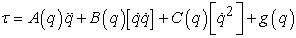(1)

Where:

q: is the joint position (joint angle).

A (q): is the n * n kinetic energy matrix, which is symmetric.

B (q): is the n * n (n-1)/2 matrix of Coriolis torques.

C (q): is the n *n matrix of centrifugal torques.

g (q):is the n-vector of gravity torques;: is the n-vector of accelerations.: is the generalized joint force vector.

The symbolsandare respectively the notation for the n*(n-1)/2 vector of velocity products and the n*1vector of squared velocities, given by:(2)The matrices A (q), B (q), C (q) and g (q) are given in following  with the Abbreviation used: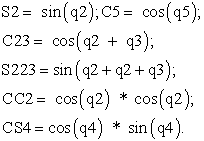(3)

The expressions giving the elements of the kinetic energy matrix A, having the units of kg-m2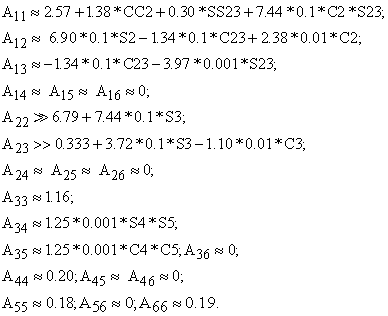(4)

The expressions giving the elements of the Coriolis matrix B, having the units of kg-m2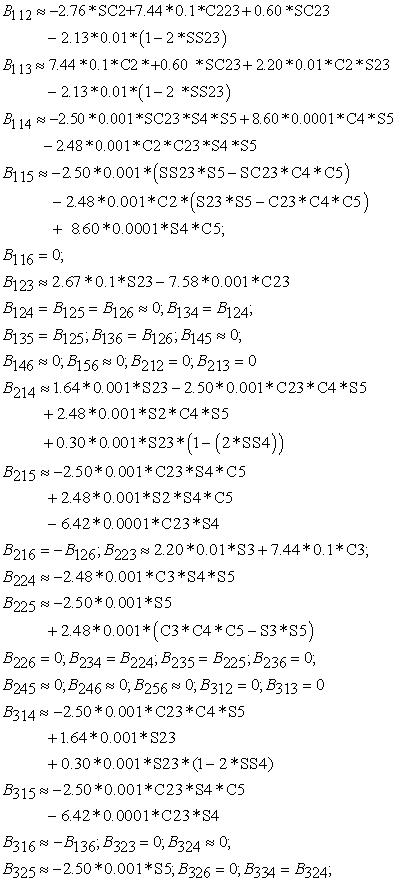(5)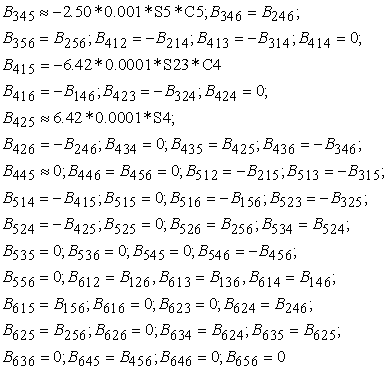The expressions giving the elements of the Coriolis matrix C, having the units of kg-m2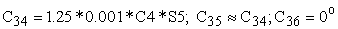(6)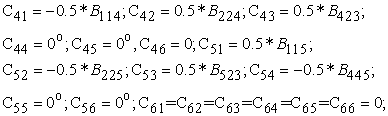Gravity terms, having the units of Newton-meters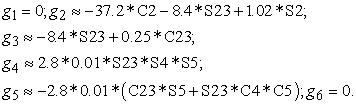(7)

The direct dynamic model (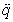) given in  used to simulate the behavior of our arm manipulator and its control loop, is given:(8)

So to simulate the behavior of our arm as indicate the Figure 3 in , we need several blocks like the trajectory generator block and the control block.

3.3. Trajectory Generation

The motion can be described both in joint space and Cartesian space as described in .in our work, we consider methods of path generation in which the path shapes are described in terms of functions of joint angles.

Many ways to generate a trajectory in the joint space in  and , among them the fifth order polynomial given by the following equation: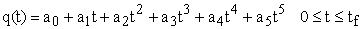(9)

And since in :With: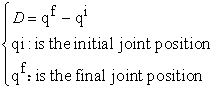Then:(10)
3.4. The Computed Torque Controller

Although control systems based on approximate linear models are popular in current industrial robots, it is important to consider the complete nonlinear dynamics of the manipulator when synthesizing control algorithms . Computed torque controller is based on feedback linearization and computes the required arm torques using the nonlinear feedback control law , this nonlinear technique of controlling a manipulator promise better performance than do simpler linear schemes.

We wish to develop this control in the configuration space, under the assumption of that the joint position and velocities are measurable and that the measurements are not affected with noise.

We have chosen the computed control law given in  in case that the motion is completely specified (i.e. we wish to cause the manipulator joints to follow prescribed position trajectories), one possibility is to use the dynamic equation of the arm as follow: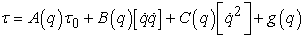(11)

Where(12),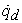, and: Are respectively the desired joint accelerations, velocities and positions in the joint space, calculates by the trajectory generator.: Is the generalized joint force vector: Is the auxiliary control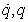: Are respectively the joint velocities and positions determined by using feedback from the joint sensors to compute the torques required.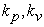: Are controller gains

4. NSGAII Controller Analysis, Modeling and Implementation on PUMA 560 Arm Manipulator

4.1. PD Controllers

The block diagram shown in the Figure 7 illustrates a closed-loop system with a PD controller in the direct path, which is the usual connection. The system's output should follow as closely as possible the reference signal (set point). The PD controller is characterized by two gains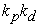, as shown in Figure 8 wherecontroller gains.

4.2. NSGA-II Algorithm

Non-dominated Sorting Genetic Algorithm II (NSGA-II) is the heavily revised version of the Non-dominated Sorting Genetic Algorithm (NSGA), which was introduced in the mid 1990 . The NSGA algorithm is a popular non-domination based genetic algorithm for multi-objective optimization. It is a very efficient algorithm but has been generally criticized for its computational complexity, needs elitism operator and a value of the sharing parameter ‘σ share’ should be chosen a priori .The NSGA-II is a modified version that adopts a more efficient ranking procedure than its predecessor . Also, it estimates the density of solutions surrounding a particular solution in the population by computing the average distance of two points on either side of this point along each of the objectives of the problem. This value is the so-called crowding distance. During selection, the NSGA-II uses a crowded -comparison operator which takes into consideration both the non domination rank of an individual in the population and its crowding distance (i.e., non-dominated solutions are preferred over dominated solutions, but between two solutions with the same non domination rank, the one that resides in the less crowded region is preferred) .

4.2.1. General Description of NSGA-II

1. The population is initialized as usual.

2. The m fitness values of the individuals in the population are calculated.

3. The rank of each individual (solution) in the population is calculated based in Pareto dominance relation originally proposed by Francis Ysidro Edgeworth in 1881,but generalized by the French Italian economist Vilfredo Pareto in 1896 . The set of Pareto optimal solutions is defined in the following definitions:

Definition: The Pareto optimal set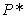is defined as: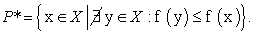(13)

4. The first front being completely non-dominant set in the current population and the second front being dominated by the individuals in the first front only and the front goes so on. Each individual in each front are assigned rank (fitness) based on front in which they belong to. Individuals in first front are given a value of 1 and individuals in second are assigned fitness value as 2 and so on.

5. Calculate the crowding distance, a new parameter, calculating as follow for each individual :

For each front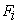, n is the number of individuals.

•  Initialize the distance to be zero for all the individuals i.e.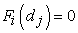, where j corresponds to theindividual in front Fi.

•  For each objective function m

*Sort the individuals in frontbased on objective m i.e.* Assign infinite distance to boundary values for each individual ini.e.* For k=2 to (n-1)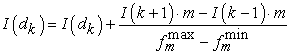(14)

Where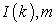is the value of the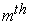objective function of the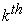individual in I.

6. The parents for the crossover are selected: Parents are selected from the population by using binary tournament selection based on the rank and crowding distance. An individual is selected if the rank is lesser than the others. In case of solutions that have the same rank, the individual with the greatest crowding distance is chosen.

7. Generation of the off springs: The selected individuals generate off springs from crossover and mutation operators.

8. The old population and current offspring are sorted again based in non-domination, and only the best N individuals are selected, where N is the population size. The selection is based in rank and the crowding distance on the last front.

9. Back to 6 a convergence criterion is met. More details on the algorithm are found in .

4.3. NSGAII Tuning of PD Controller of Puma560

The NSGAII algorithm is based on the genetic algorithm, so to represent a solution we must define it as a chromosome (i.e.: a string). Puma560 contains independent controller for each joint. For six joint controllers, there are 12 values for PD parameters.

By taking a 12 variable string asfor NSGAII, an optimal value can be searched. The method of tuning PD parameters using NSGAII is based in minimizing the Integral of Absolute value of Error IAE and considerate as vector of fitness of joints . If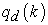is desired trajectory vector andis output trajectory vector then the error vector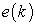is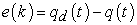(15)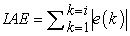(16)

Whereis the system error atsampling instant.

The implementation of the NSGAII is presented as follow:

1. Population is initialized

This function initializes the population with N individuals. Each individual is presented as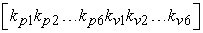, and eachtaken its value randomly from2. Each fitness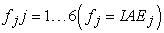is calculated for each individual in the population, and save its value, by concatenation of each individual string and its fitness string.

3. Sort the current population based on the non-domination sort by calculating the rank value and the crowding distance value of each individual.

4. Individuals (parents) are selected by tournament selection.

5. The Simulated Binary Crossover (SBC) is applied to generate off springs find in . In addition to the crossover and mutation probabilities, the SBC needs to be implemented two new parameters: the distribution index for crossover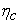and the mutation distribution index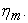6. Step 2 and 3 are applied to the whole population

7. Next population is formed by selection of the best n individuals having minimum rank, and the greater crowding distance for the individual of last rank.

8. back to 4 until a predefined number of generations.

NSGAII acts as a controller which modifies the set of parameters of the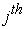population which consist of P individual parameters of control system. This modification is based in minimizing simultaneously six position errors.

In the evaluation step of NSGAII, a simulation is performed for each controller. Parameters used for simulation of NSGAII given in  are: Crossover probability < 0.9, Mutation probability > 0.9 ,,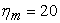, tour-size=2 (size of the tournament selection), pool-size=ceil (size (population, 1)/2): pool-size is the number of individuals selected to form a mating pool after performing tournament selection.

The population size and the generation number are defined by the user.

5. Results and Discussion

The simulation was implemented in MATLAB/ SIMULINK environment. It is noted that, to simulate the puma560 model in the Simulink environment, we used the PD values given in Table.1, found empirically in .

Table 1. PD gains

To generate trajectory in joint space directly, we used as initial and final positions noted respectively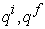found in :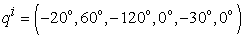(17)(18)

The system is simulated in 1 second and the sampling time is 0.01s.

5.2. Result of NSGAII Algorithm Using for PD Tuning

In the experiments, the MATLAB R2012a environment has been used for implementing and running the NSGAII algorithm. Experiments with PUMA560 system have been performed for the evaluation of the tuning procedure.

The results obtained are the joint angles (joint positions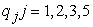) and the joint velocities (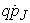, which are respectively, compared with the desired joint angles values and the desired joint velocities values. We don’t cite the 4th and the 6th joint because it goes from 0° to 0°.

As a test, the NSGAII algorithm has been configured as follows: Population size: 2, Generations: 2. Results are shown in Figure 17 to Figure 24 with an elapsed time 1.4534 s.

It can be seen in Figure 17 to Figure 24 that the NSGAII joint angles curves converge to the desired joint angles curves. Also, it can be observed that the NSGAII joint velocities curves converge to the desired joint velocities curves with very small deviation in some areas of the velocities curves.

The Figure 25 shows that in 0.71s and 0.72 second all fitness values i.e. the IAE (0.71) are nearly equal to IAE (0.72) as shown in table.2, that is to say that the errors in that seconds are near zero in 0.72s, so we can say that the PD gains generated in this time are good for the six joint controllers.

Table 2. IAE error of the 1st,2nd ,3rd and the 5th joint of thePUMA560

From the table.2, we can say that the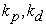gains generated at the 0.72s are the best, as shown in table.3:

Table 3. The best PD gains obtained by NSGAII

Results of model simulations where the gains are tuned empirically Figure 9 to Figure 16 are very good, but because some joints P gain are high (700, 1100) compared to those obtained numerically by NSGAII that are between 0 and 100, we can say that tuning through NSGAII led to satisfactory responses for the system tested because in , in industry we cannot use regulators with so huge gains.

The Figure 26 shows the absolute error of the joint angles obtained by minimizing the IAE errors.

So the NSGAII tuned PD controller is showing good performance in 1.4534 s

6. Conclusion

This work is focused on the NSGAII (Non-dominated Sorting Genetic Algorithm II) algorithm and investigation of its applicability to the automatic tuning of PD controllers of the PUMA560 system. And since the system has six PD controllers, the method searches for a combination of gains so that the errors between actual and desired responses are minimized. The method was carried out in term of IAE in joint space. It can be concluded from the results that NSGAII is showing good results. As sometimes is the case with this method, there was no need for further manual adjustments to the PD gains when automatic tuning was employed.

References

  Whidborne, J.F., G. Murad, D.-W. Gu and I. Postlethwaite Robust control of an unknown plant, the IFAC 93 benchmark. Int. J. Control, vol. 61, no. 3, pp. 589-640, 1995a.In article CrossRef  Whidborne, J. F., I. Postlethwaite and D.-W. Gu A mixed optimization approach to multi-objective computer-aided control system design, In Proc. IEEE Symp. on Comp. Aided Contr. Syst. Design (CACSD'96). Dearborn, Michigan: pp. 309-314, 1996.In article  Liu, G. P. and R. J. Patton, Parametric state feedback controller design of multivariable systems, International Journal of Control, vol. 61, no. 6, pp. 1457-1464, 1995.In article CrossRef  Liu, G. P. and R. J. Patton, Robust control design using eigenstructure assignment and multiobjective optimization, Int. Journal of Systems Science, vol. 27, no. 9, pp. 871-879, 1996.In article CrossRef  Whidborne, J.F., I. Postlethwaite and D.-W. Gu, Multiobjective control system designs a mixed optimization approach, Recent Trends in Optimization Theory and Applications, R. P. Agarwal (ed.), vol. 5 of World Scientificc Series in Applicable Analysis Singapore: World Scientific pp. 467-482, 1995b.In article  Liu, G. P. and S. Daley, Stable dynamical controller designs for robust polynomial pole assignment,. IEE Proceedings, Part D, vol. 145, no. 3, pp. 259-264, 1998.In article  Liu, G. P. and S. Daley, Optimal-tuning PID control for industrial systems, Control Engineering Practice, vol. 9, 2001 (accepted).In article CrossRef  Liu, G. P. and V. Kadirkamanathan, Multiobjective criteria for nonlinear model selection and identification with neural networks, IEE Proceedings, Part D, vol. 146, no. 5, pp. 373-382, 1999.In article  Chen, J., R. J. Patton and G. P. Liu, Optimal residual design for fault diagnosis using multi-objective optimisation and genetic algorithms, International Journal of Systems Science, vol. 27, no. 6, pp. 567-576, 1996.In article CrossRef  Tabak, T., A. A. Schy, D. P. Giesy, and K. G. Johnson, Application of multiple objective optimization in aircraft control systems design,. Automatica, vol. 15, pp. 595-600. 1979.In article CrossRef  Skelton, R. E. and M. DeLorenzo, Spacestructure control design by variance assignment, J, Guid., vol. 8, no. 4, pp. 454-462. 1985.In article CrossRef  Koussoulas, N. T. and C. T. Leondes, The multiple linear quadratic Gaussian problems. International Journal of Control, vol. 43, no. 2, pp. 337-349. 1986.In article CrossRef  Toivonen, H. T. and P. M. Makila, Computer-aided design procedure for multi-objective LQG control problems, International Journal of Control, vol. 49, no. 2, pp. 655-666. 1989.In article  Khargonekar, P. P. and M. A. Rotea, Multiple objective optimal control of linear systems: the quadratic norm case,IEEE Trans. Automat. Contr., vol. 36, no.1, pp. 14-24, 1991.In article CrossRef  Jaimes A.L., Zapotecas Martinez S., C.A. C. Coello, Chapter 1 An Introduction To Multi-Objective Optimization Techniques, In: Book Title Editor: Editor Name, pp. 1-26, 2009 Nova Science Publishers, Inc.In article  Gambier A, MPC and PID Control Based on Multi-objective Optimization, American Control Conference Westin Seattle Hotel, Seattle, Washington, USA, June 11-13, 2008.In article  Zitzler E and Thiele L, Multiobjective evolutionary algorithms: a comparative case study and the strength Pareto approach. IEEE Transactions on Evolutionary Computation, 1999, 3(4):257-271.In article CrossRef  J. Rutherford, Using the PUMA560 Robot, PUMA Unofficial User’s Guide, 2012.In article  J. J. Craig, Introduction to Robotics Mechanics and Control, Third Edition” 2005 Pearson Education, Inc.In article  B Armstrong, O Khatib, Joel Burdick, The Explicit Dynamic Model and Inertial Parameters of the PUMA 560 Arm, IEEE, 1986.In article  W. khalil, E. Dombre, Modélisation et commande des robots, Hermes Edition, Paris, 1988.In article  J. J. Craig, Introduction to Robotics Mechanics and Control, second Edition, 1989, 1986 by Addison-Wesley Publishing Company, Inc.In article  F. Piltan , M.H. Yarmahmoudi, M. Shamsodini, E. Mazlomian & A. Hosainpour, PUMA-560 Robot Manipulator Position Computed Torque Control Methods Using MATLAB/SIMULINK and Their Integration into Graduate Nonlinear Control and MATLAB Courses, International Journal of Robotics and Automation, (IJRA), Volume (3): Issue (3) : 2012.In article  D. Czarkowski, Identification And Optimization PID Parameters Using Matlab, report of the project for bachelor of engineering in electronics, Cork Institute of Technology Gdynia Maritime University, Cork 2002.In article  Sumathi S, Surekha P, Computational intelligence paradigms: theory & applications using MATLAB, © 2010 by Taylor and Francis Group, 2010.In article  N. Moreira , P. Alvito and P. Lima, First steps towards an open control architecture for a PUMA 560, Proc. 2nd Portuguese Conf. On Automatic Control, 1996.In article